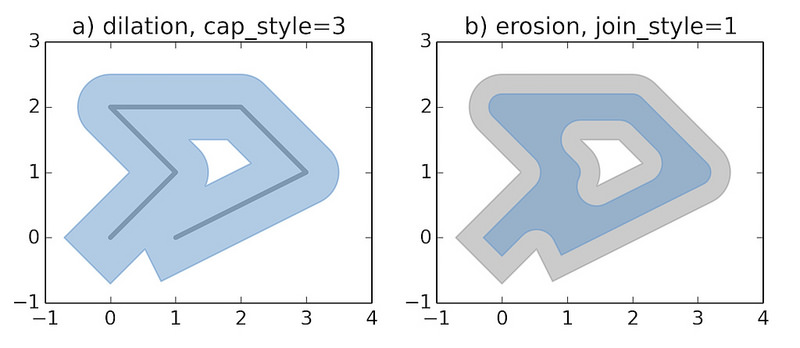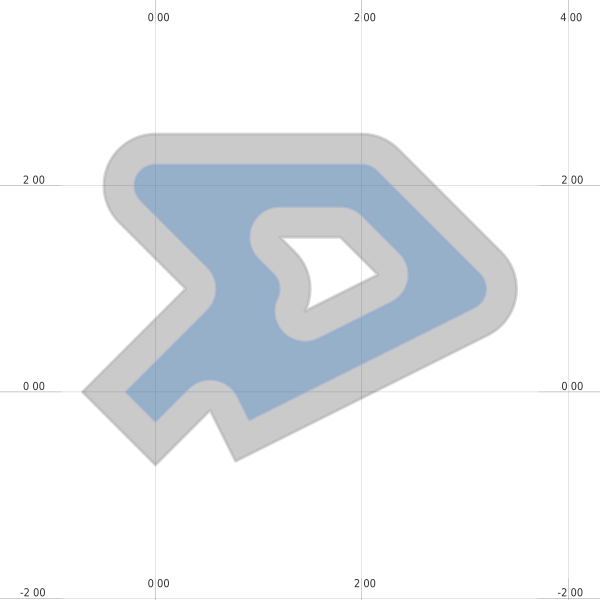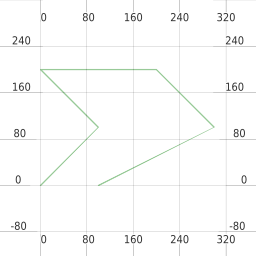# 7. Integrating with Python¶

This page gives some examples of integrating mappyfile into Python based work flows.

## 7.1. Displaying Geometry¶

The examples below recreates the sample images used in the Shapely documentation.```mapfile["size"] = [600, 600]
```

### 7.1.1. Dilated¶

```def dilation(mapfile):

line = LineString([(0, 0), (1, 1), (0, 2), (2, 2), (3, 1), (1, 0)])
ll = mappyfile.find(mapfile["layers"], "name", "line")
ll["features"]["wkt"] = line.wkt

dilated = line.buffer(0.5, cap_style=3)
pl = mappyfile.find(mapfile["layers"], "name", "polygon")
pl["features"]["wkt"] = dilated.wkt

mapfile["extent"] = " ".join(map(str, dilated.buffer(0.8).bounds))
return dilated
```### 7.1.2. Eroded¶

```def erosion(mapfile, dilated):
"""
We will continue to work with the modified Mapfile
If we wanted to start from scratch we could simply reread it
"""
ll = mappyfile.find(mapfile["layers"], "name", "line")
ll["status"] = "OFF"

pl = mappyfile.find(mapfile["layers"], "name", "polygon")

# make a deep copy of the polygon layer in the Map
# so any modification are made to this layer only
pl2 = deepcopy(pl)

pl2["name"] = "newpolygon"
mapfile["layers"].append(pl2)

dilated = dilated.buffer(-0.3)
pl2["features"]["wkt"] = dilated.wkt

style = pl["classes"]["styles"]
style["color"] = "#999999"
style["outlinecolor"] = "#b2b2b2"
```